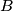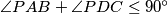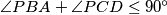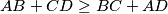### IMO Shortlist 2006 problem G8

Kvaliteta:
Avg: 0,0
Težina:
Avg: 9,0
Let$ABCD$ be a convex quadrilateral. A circle passing through the points$A$ and$D$ and a circle passing through the points$B$ and$C$ are externally tangent at a point$P$ inside the quadrilateral. Suppose that$\angle{PAB}+\angle{PDC}\leq 90^\circ$ and$\angle{PBA}+\angle{PCD}\leq 90^\circ$.
Prove that$AB+CD \geq BC+AD$.
Izvor: Međunarodna matematička olimpijada, shortlist 2006